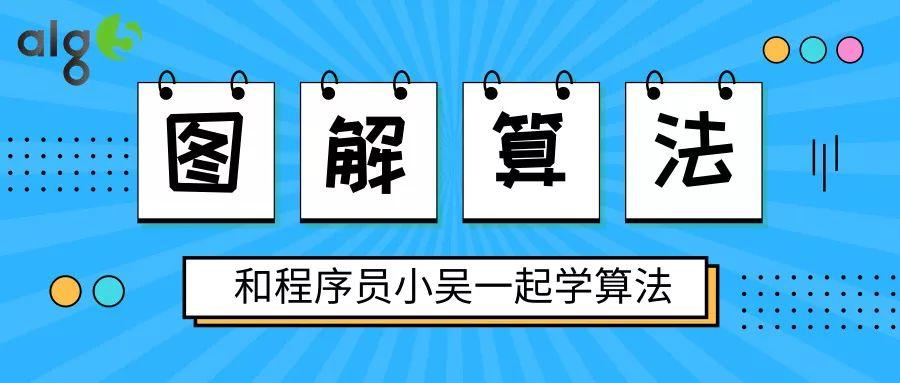### 题目描述

输入: [2,2,3,4]输出: 3解释:有效的组合是: 2,3,4 (使用第一个 2)2,3,4 (使用第二个 2)2,2,3

• 数组长度不超过1000。

• 数组里整数的范围为 [0, 1000]。

### 题目解析

a < b < c && a + b > c => 三角形

### 代码实现

public int triangleCount(int[] S) {    if (S == null || S.length == 0) {        return 0;    }    Arrays.sort(S);    int result = 0;    for (int i = S.length - 1; i >= 2; --i) {        int l = 0, r = i - 1;        while (l < r) {            if (S[i] < S[l] + S[r]) {  // S[i] < S[l] + S[r] && S[i] > S[r] > S[l]                result += r - l;  //  直接加上可能的个数                r--;            } else {                l++;            }        }    }    return result;}1.程序员

2.【GitHub

3.【算法动画：七分钟理解什么是KMP算法

4.【数据结构十大经典排序算法动画与解析，看我就够了！